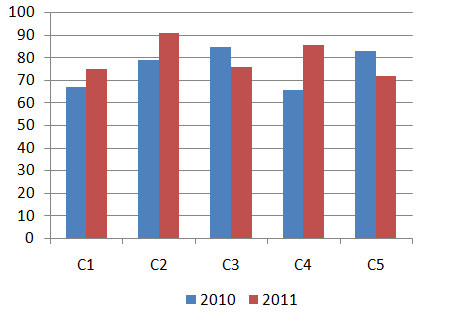Question 100

# Directions:Study the following bar graph and answer the question that follows:Sales of books (in thousands) from 5 (C1,C2,C3,C4 and C5) branches of a publishing company for the two successive years 2010 and 2011.What is the average sales of all the branches (in thousands) for the year 2010?Solution

Total sales of all the branches (in thousands) for the year 2010

= 68 + 79 + 85 + 66 + 82 = 380

=> Average sales of all the branches (in thousands) for the year 2010 = $$\frac{380}{5}=76$$

=> Ans - (D)